# Chapter 10 Chemical Quantities 10 1 The Mole

• Slides: 76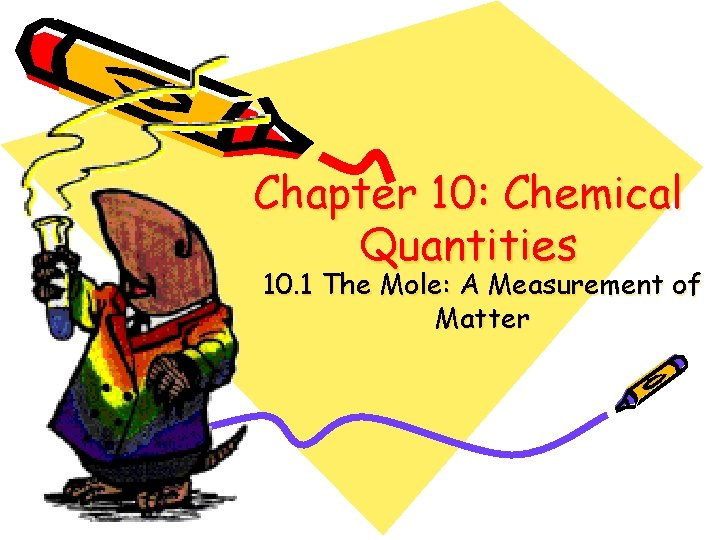Chapter 10: Chemical Quantities 10. 1 The Mole: A Measurement of Matter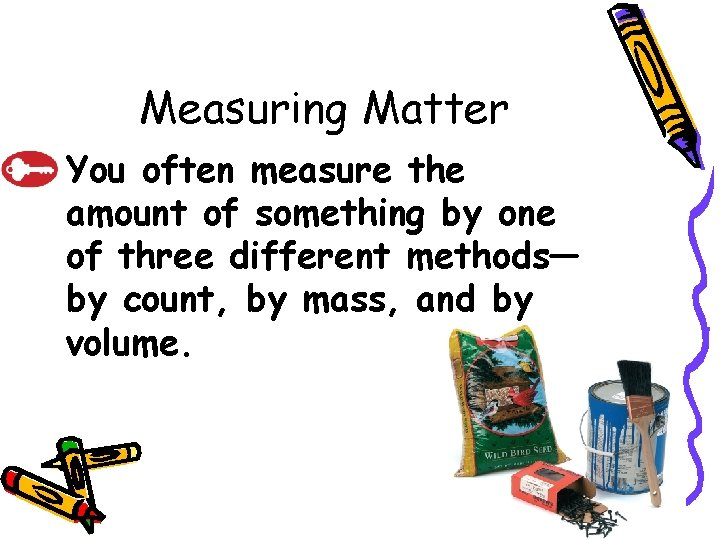10. 1 Measuring Matter – You often measure the amount of something by one of three different methods— by count, by mass, and by volume.Sample Problem 10. 1for Sample Problem 10. 1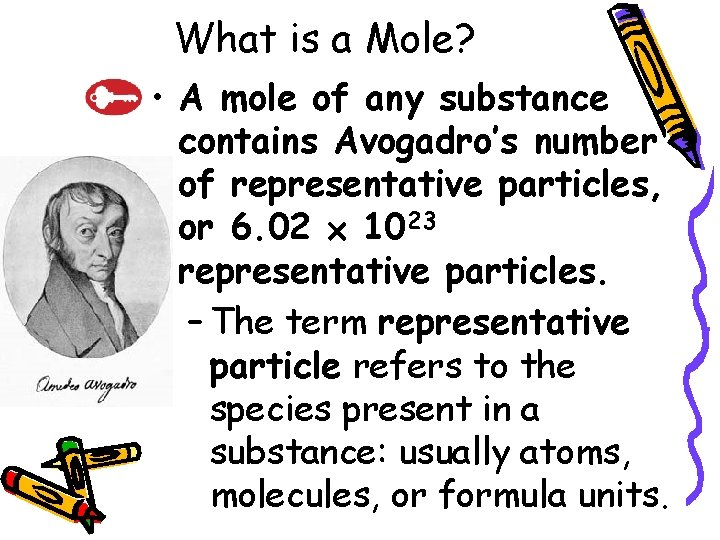10. 1 What is a Mole? • A mole of any substance contains Avogadro’s number of representative particles, or 6. 02 1023 representative particles. – The term representative particle refers to the species present in a substance: usually atoms, molecules, or formula units.10. 1 • Converting Number of Particles to Moles – One mole (mol) of a substance is 6. 02 1023 representative particles of that substance and is the SI unit for measuring the amount of a substance. – The number of representative particles in a mole, 6. 02 1023, is called Avogadro’s number.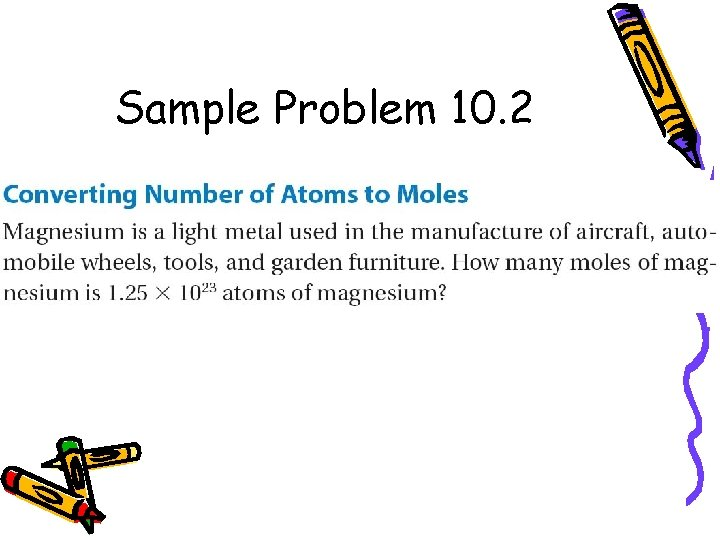Sample Problem 10. 2for Sample Problem 10. 2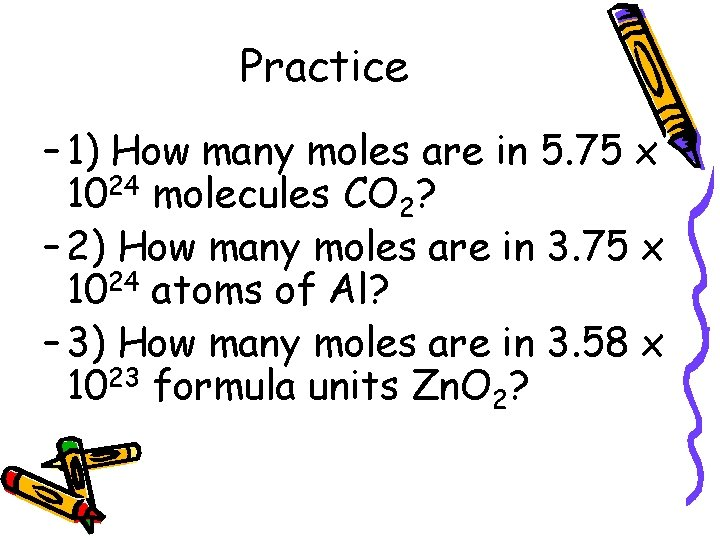Practice – 1) How many moles are in 5. 75 x 1024 molecules CO 2? – 2) How many moles are in 3. 75 x 1024 atoms of Al? – 3) How many moles are in 3. 58 x 1023 formula units Zn. O 2?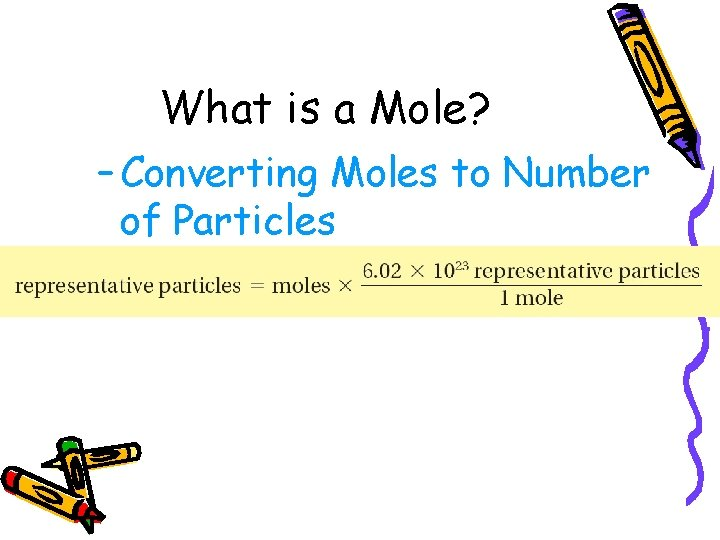10. 1 What is a Mole? – Converting Moles to Number of Particles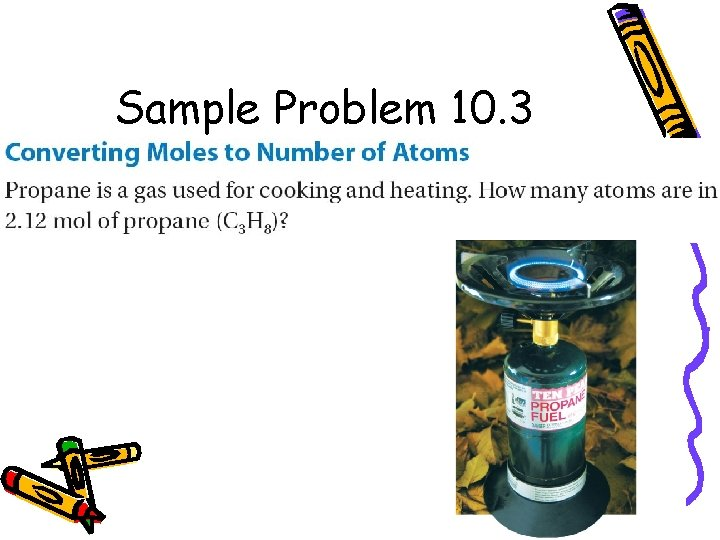Sample Problem 10. 3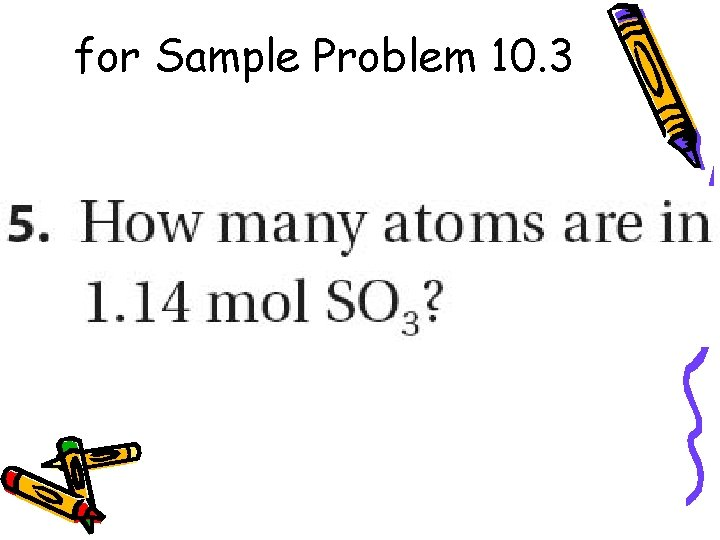for Sample Problem 10. 3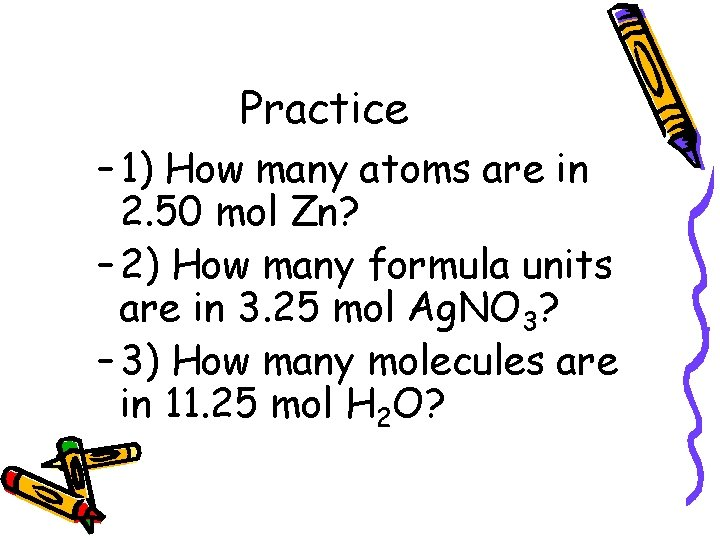Practice – 1) How many atoms are in 2. 50 mol Zn? – 2) How many formula units are in 3. 25 mol Ag. NO 3? – 3) How many molecules are in 11. 25 mol H 2 O?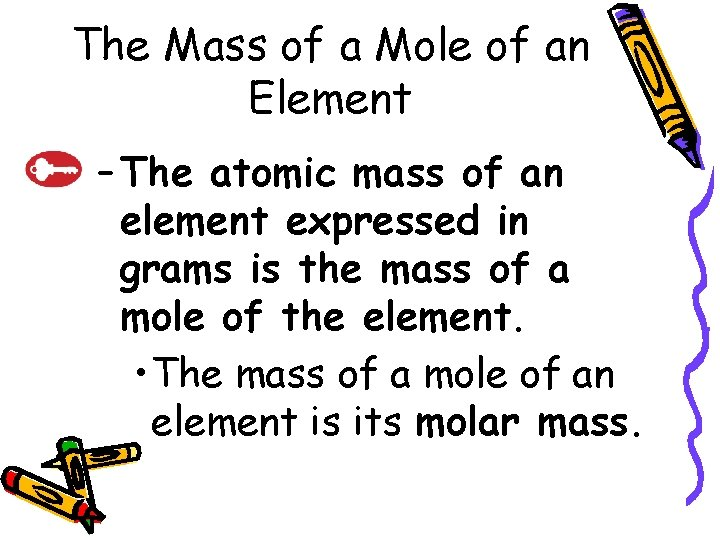10. 1 The Mass of a Mole of an Element – The atomic mass of an element expressed in grams is the mass of a mole of the element. • The mass of a mole of an element is its molar mass.B. Molar Mass Examples – carbon – aluminum – zinc10. 1 The Mass of a Mole of a Compound – To calculate the molar mass of a compound, find the number of grams of each element in one mole of the compound. Then add the masses of the elements in the compound.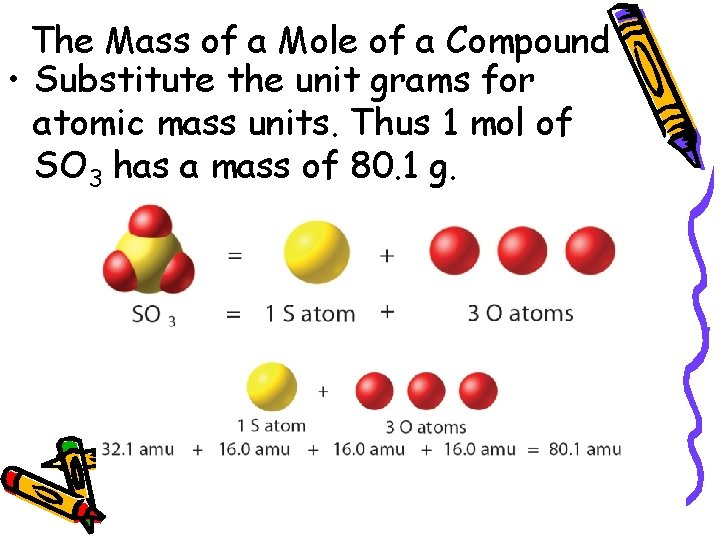10. 1 The Mass of a Mole of a Compound • Substitute the unit grams for atomic mass units. Thus 1 mol of SO 3 has a mass of 80. 1 g.Sample Problem 10. 4for Sample Problem 10. 4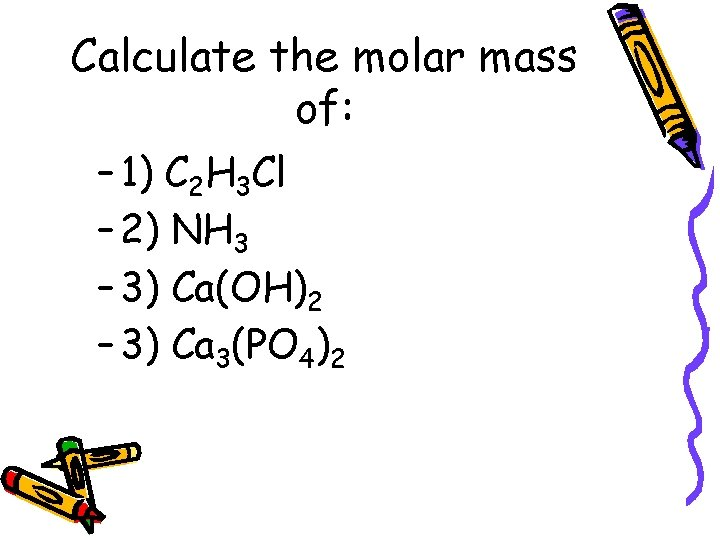Calculate the molar mass of: – 1) C 2 H 3 Cl – 2) NH 3 – 3) Ca(OH)2 – 3) Ca 3(PO 4)2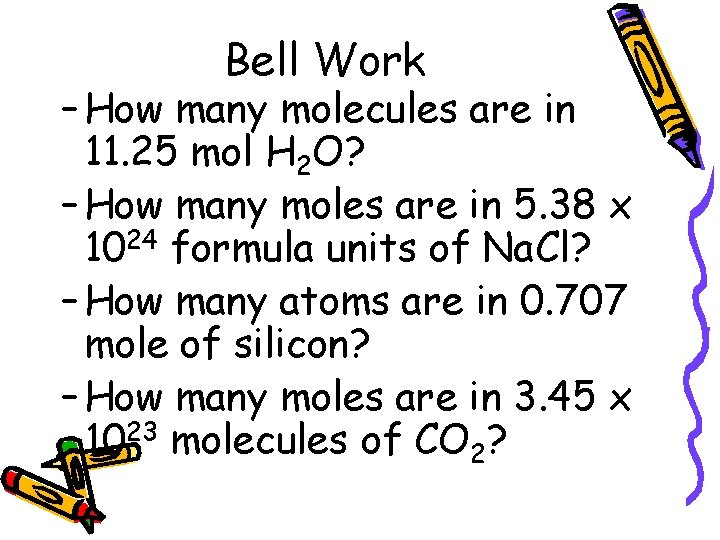Bell Work – How many molecules are in 11. 25 mol H 2 O? – How many moles are in 5. 38 x 1024 formula units of Na. Cl? – How many atoms are in 0. 707 mole of silicon? – How many moles are in 3. 45 x 1023 molecules of CO 2?10. 1 Section Quiz. – 1. Three common ways of measuring the amount of something are by count, by mass, and a) by temperature. b) by volume. c) by area. d) by density.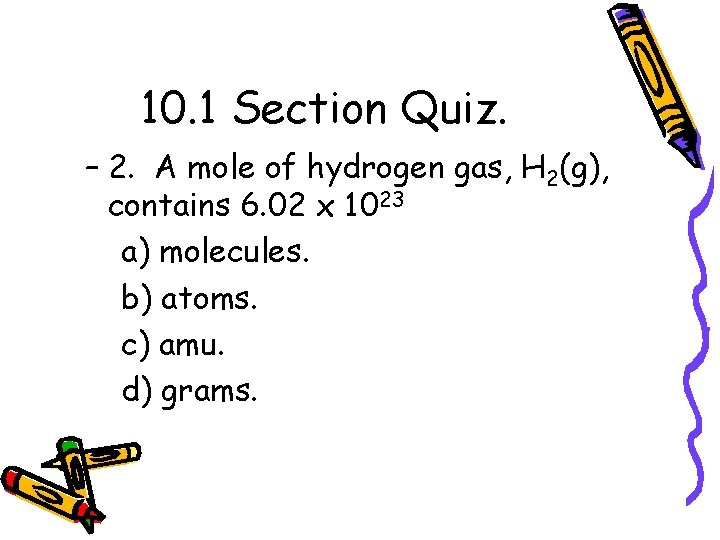10. 1 Section Quiz. – 2. A mole of hydrogen gas, H 2(g), contains 6. 02 x 1023 a) molecules. b) atoms. c) amu. d) grams.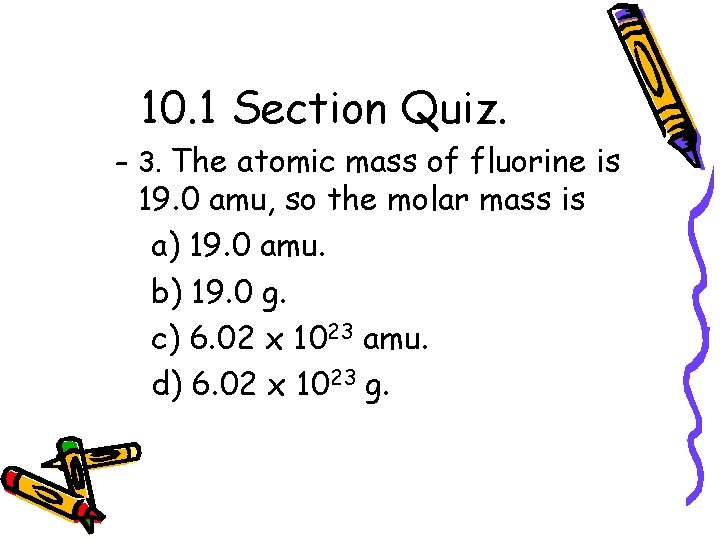10. 1 Section Quiz. – 3. The atomic mass of fluorine is 19. 0 amu, so the molar mass is a) 19. 0 amu. b) 19. 0 g. c) 6. 02 x 1023 amu. d) 6. 02 x 1023 g.10. 1 Section Quiz. – 4. Calculate the molar mass of ammonium nitrate. a) 45. 02 g b) 80. 05 g c) 60. 06 g d) 48. 05 g10. 2 Mole-Mass and Mole-Volume Relationships10. 2 The Mole–Mass Relationship – Use the molar mass of an element or compound to convert between the mass of a substance and the moles of a substance.Sample Problem 10. 5for Sample Problem 10. 5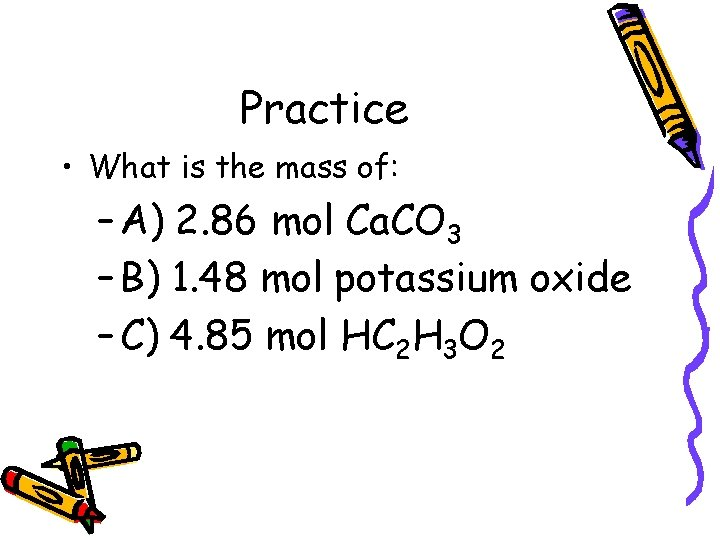Practice • What is the mass of: – A) 2. 86 mol Ca. CO 3 – B) 1. 48 mol potassium oxide – C) 4. 85 mol HC 2 H 3 O 2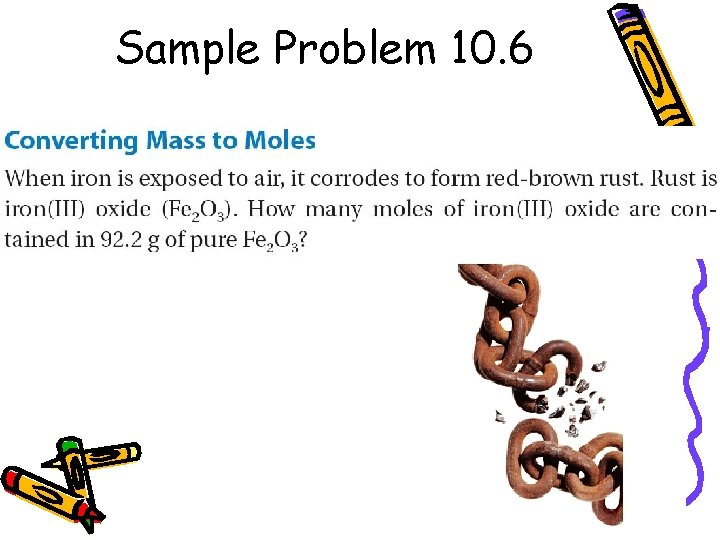Sample Problem 10. 6for Sample Problem 10. 6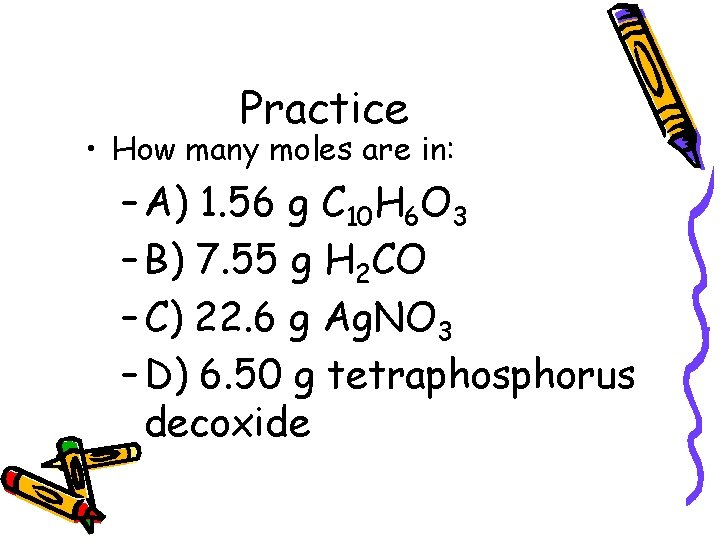Practice • How many moles are in: – A) 1. 56 g C 10 H 6 O 3 – B) 7. 55 g H 2 CO – C) 22. 6 g Ag. NO 3 – D) 6. 50 g tetraphosphorus decoxide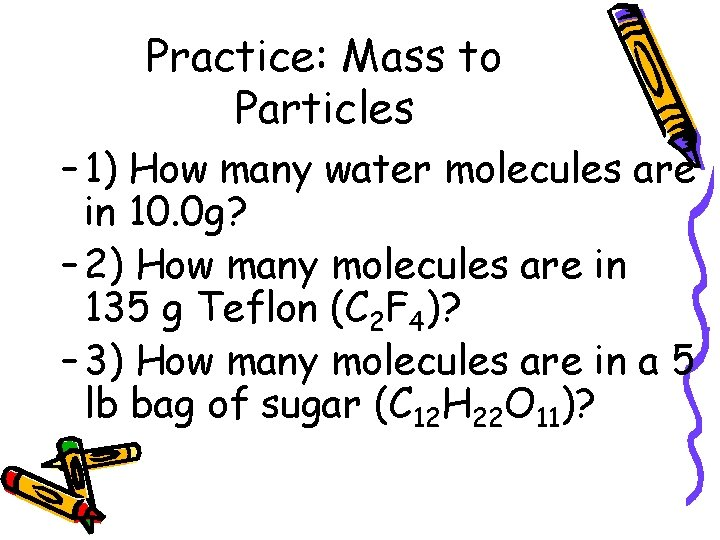Practice: Mass to Particles – 1) How many water molecules are in 10. 0 g? – 2) How many molecules are in 135 g Teflon (C 2 F 4)? – 3) How many molecules are in a 5 lb bag of sugar (C 12 H 22 O 11)?Mole Relationships from a chemical formula • Aluminum oxide, often called alumina, is the principle raw material for the production of aluminum. Alumina occurs in the minerals corundum and bauxite. Determine the moles of aluminum ions (Al 3+) in 1. 25 mol of Al 2 O 3.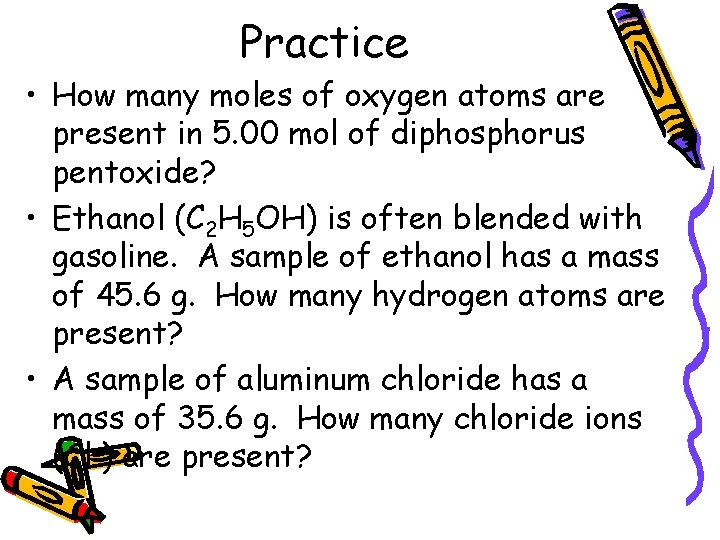Practice • How many moles of oxygen atoms are present in 5. 00 mol of diphosphorus pentoxide? • Ethanol (C 2 H 5 OH) is often blended with gasoline. A sample of ethanol has a mass of 45. 6 g. How many hydrogen atoms are present? • A sample of aluminum chloride has a mass of 35. 6 g. How many chloride ions (Cl-) are present?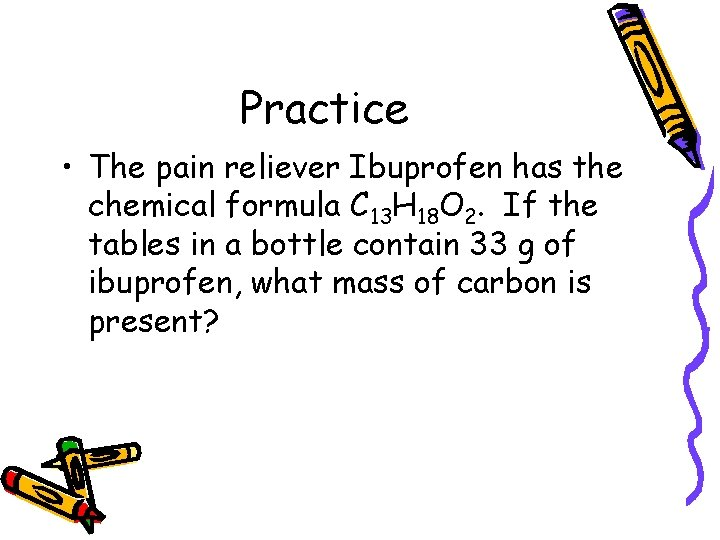Practice • The pain reliever Ibuprofen has the chemical formula C 13 H 18 O 2. If the tables in a bottle contain 33 g of ibuprofen, what mass of carbon is present?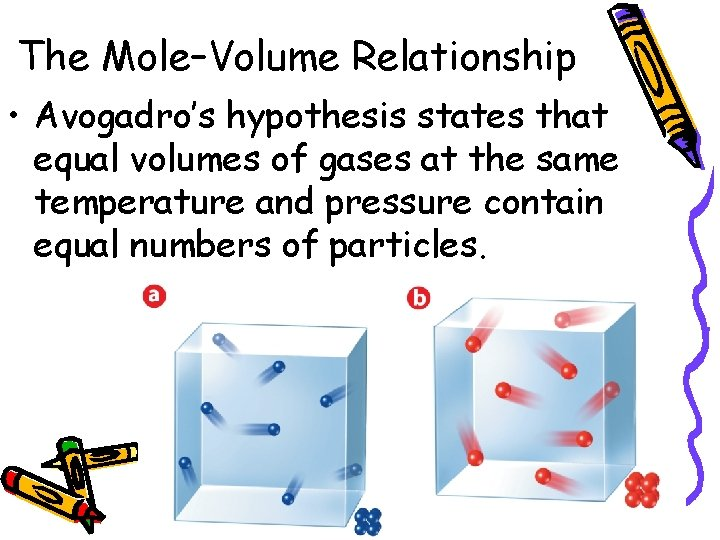10. 2 The Mole–Volume Relationship • Avogadro’s hypothesis states that equal volumes of gases at the same temperature and pressure contain equal numbers of particles.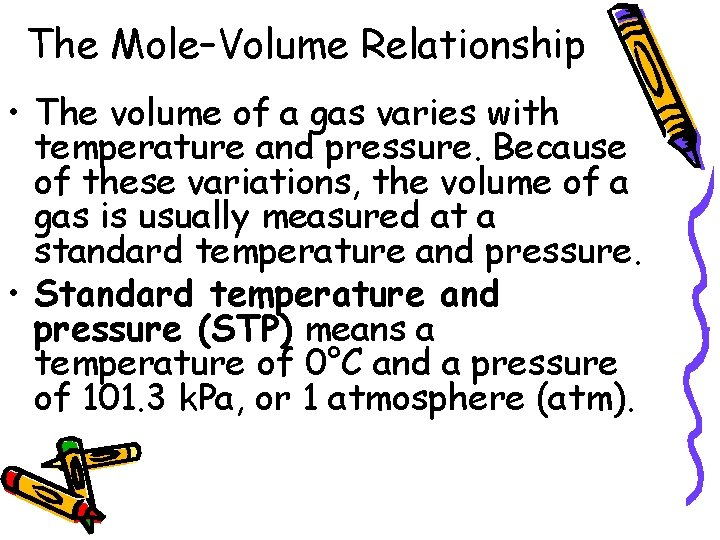10. 2 The Mole–Volume Relationship • The volume of a gas varies with temperature and pressure. Because of these variations, the volume of a gas is usually measured at a standard temperature and pressure. • Standard temperature and pressure (STP) means a temperature of 0°C and a pressure of 101. 3 k. Pa, or 1 atmosphere (atm).10. 2 The Mole–Volume Relationship • At STP, 1 mol or, 6. 02 1023 representative particles, of any gas occupies a volume of 22. 4 L. –The quantity 22. 4 L is called the molar volume of a gas.10. 2 The Mole–Volume Relationship • Calculating Volume at STP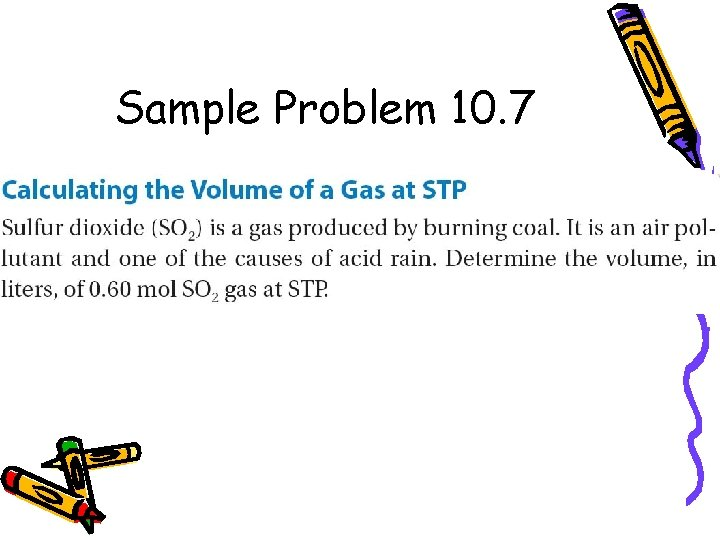Sample Problem 10. 7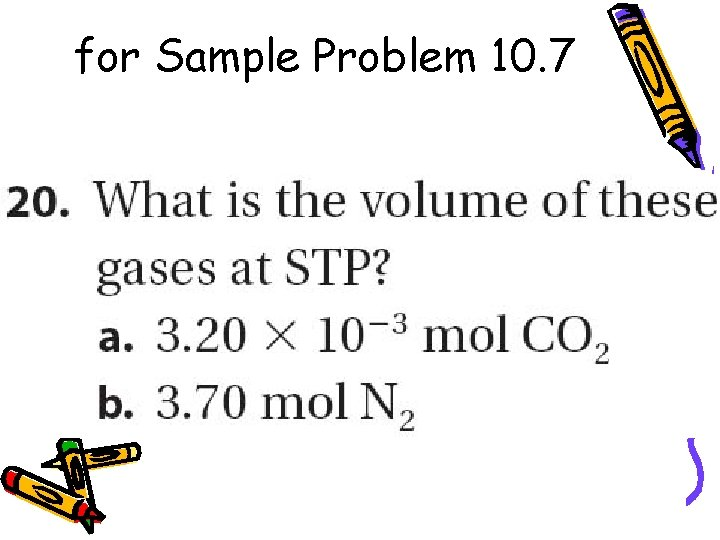for Sample Problem 10. 7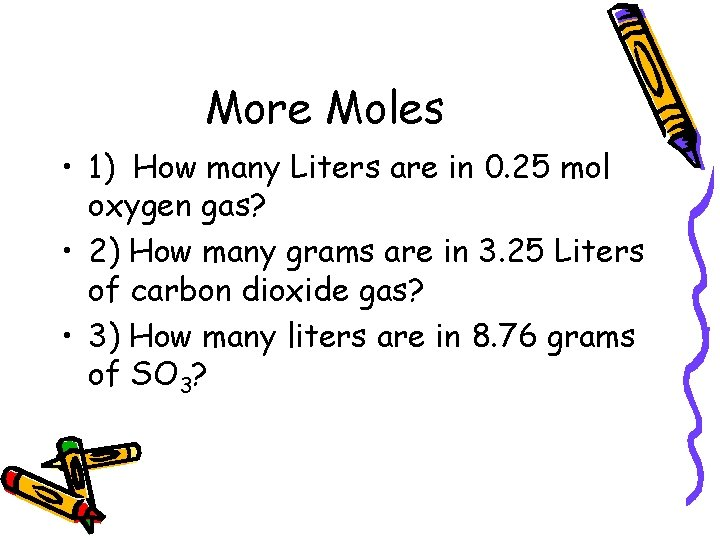More Moles • 1) How many Liters are in 0. 25 mol oxygen gas? • 2) How many grams are in 3. 25 Liters of carbon dioxide gas? • 3) How many liters are in 8. 76 grams of SO 3?10. 2 The Mole–Volume Relationship • Calculating Molar Mass from Density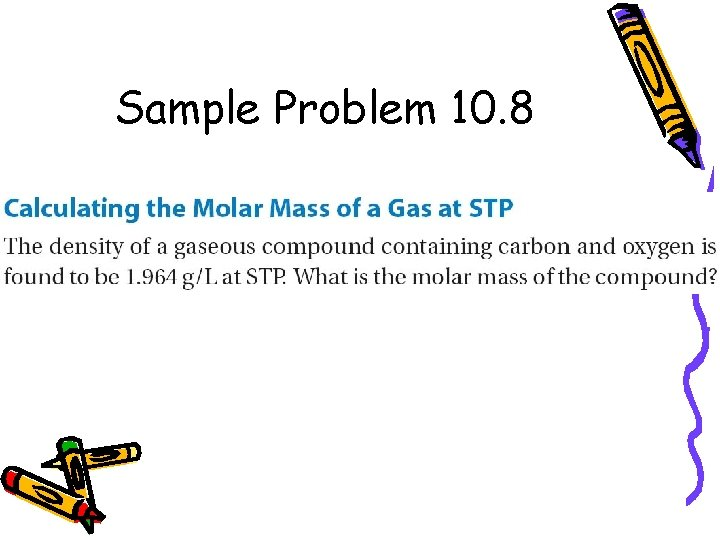Sample Problem 10. 8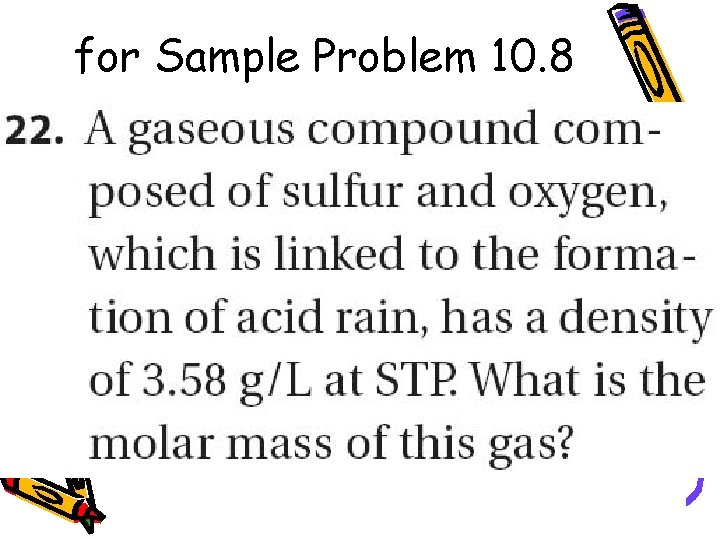for Sample Problem 10. 8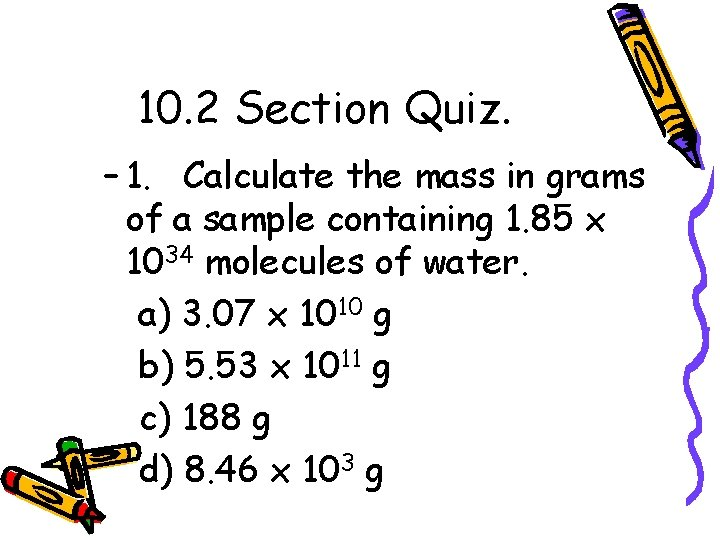10. 2 Section Quiz. – 1. Calculate the mass in grams of a sample containing 1. 85 x 1034 molecules of water. a) 3. 07 x 1010 g b) 5. 53 x 1011 g c) 188 g d) 8. 46 x 103 g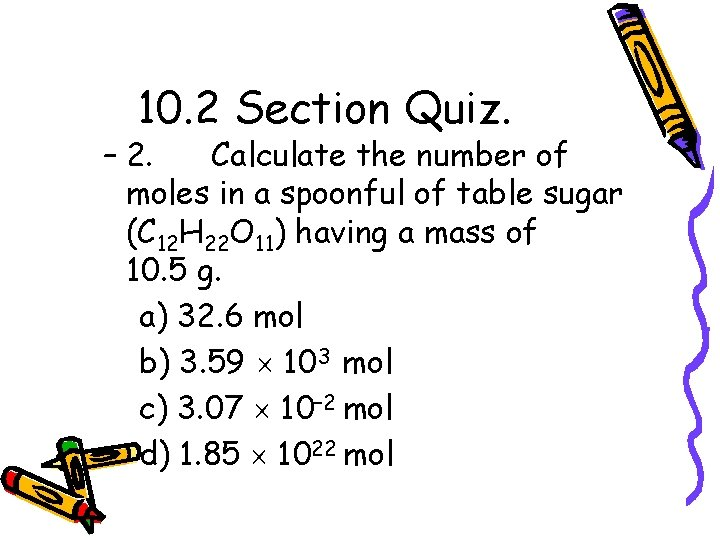10. 2 Section Quiz. – 2. Calculate the number of moles in a spoonful of table sugar (C 12 H 22 O 11) having a mass of 10. 5 g. a) 32. 6 mol b) 3. 59 103 mol c) 3. 07 10– 2 mol d) 1. 85 1022 mol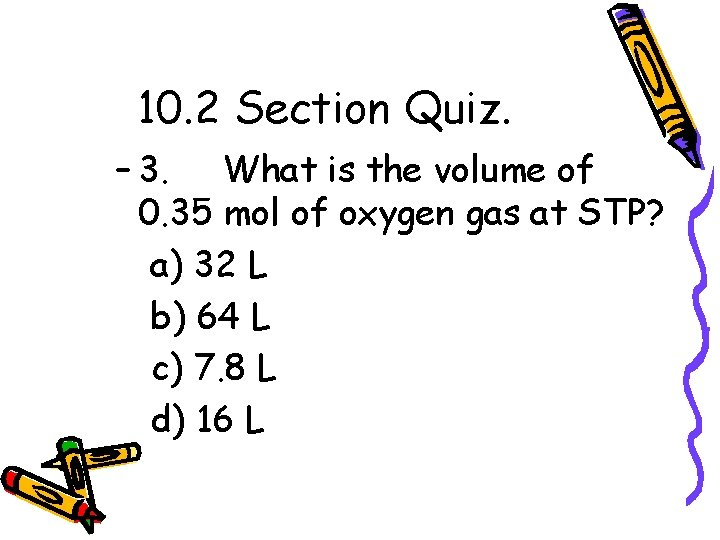10. 2 Section Quiz. – 3. What is the volume of 0. 35 mol of oxygen gas at STP? a) 32 L b) 64 L c) 7. 8 L d) 16 L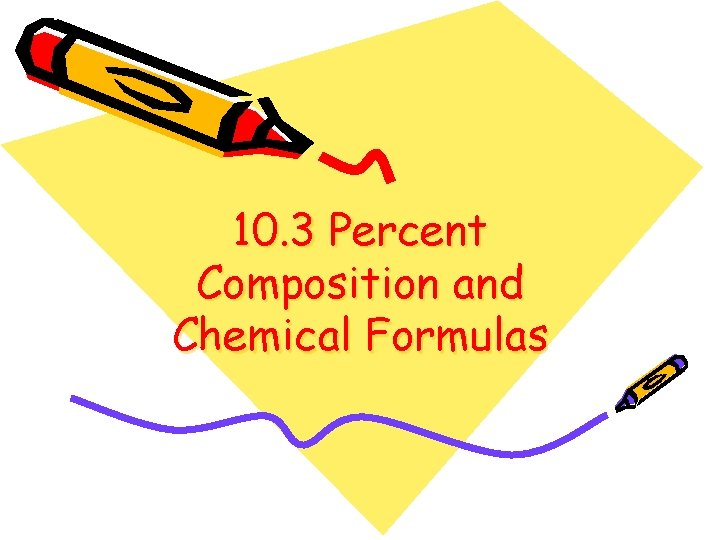10. 3 Percent Composition and Chemical Formulas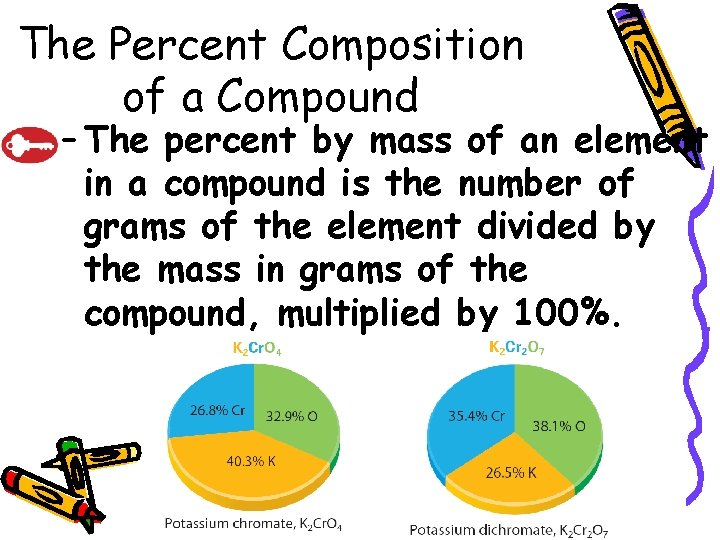10. 3 The Percent Composition of a Compound – The percent by mass of an element in a compound is the number of grams of the element divided by the mass in grams of the compound, multiplied by 100%.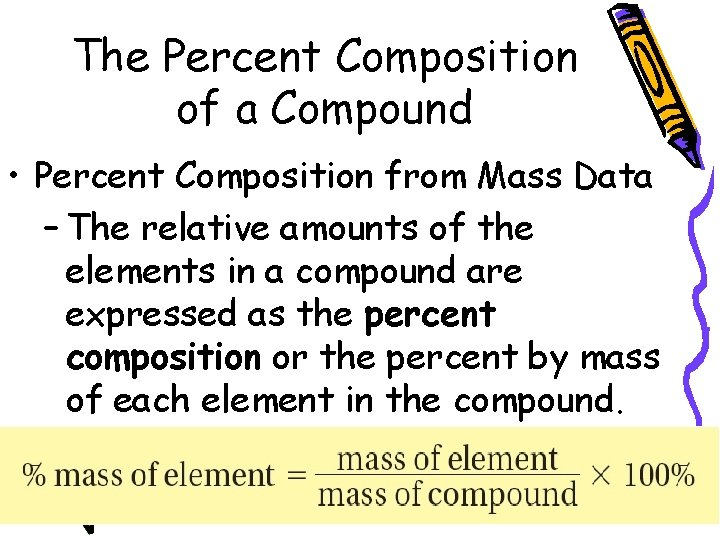10. 3 The Percent Composition of a Compound • Percent Composition from Mass Data – The relative amounts of the elements in a compound are expressed as the percent composition or the percent by mass of each element in the compound.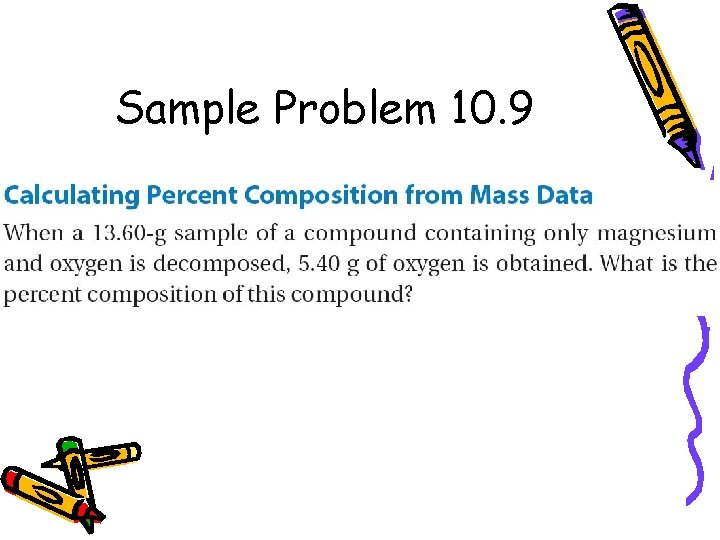Sample Problem 10. 9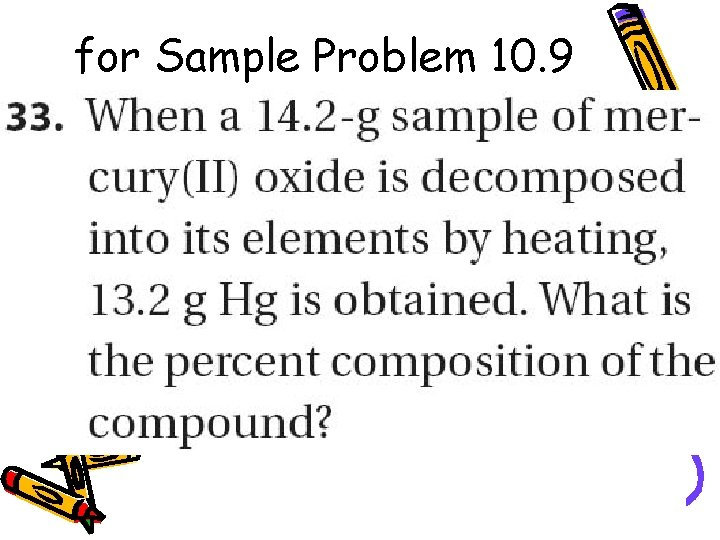for Sample Problem 10. 9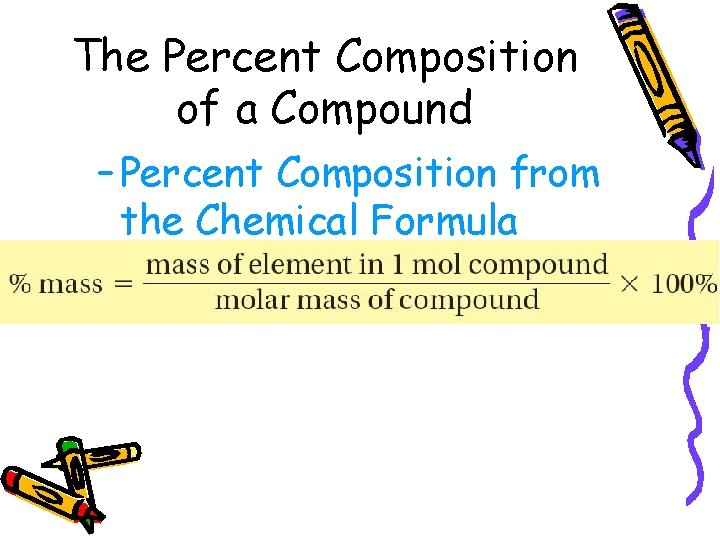10. 3 The Percent Composition of a Compound – Percent Composition from the Chemical Formula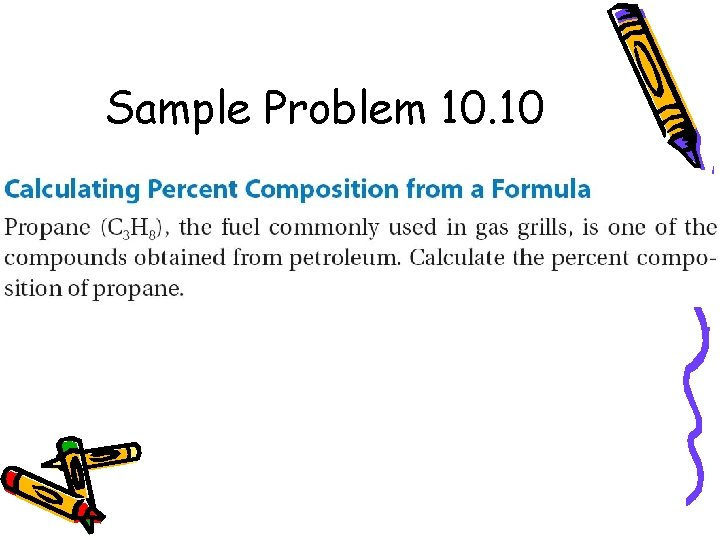Sample Problem 10. 10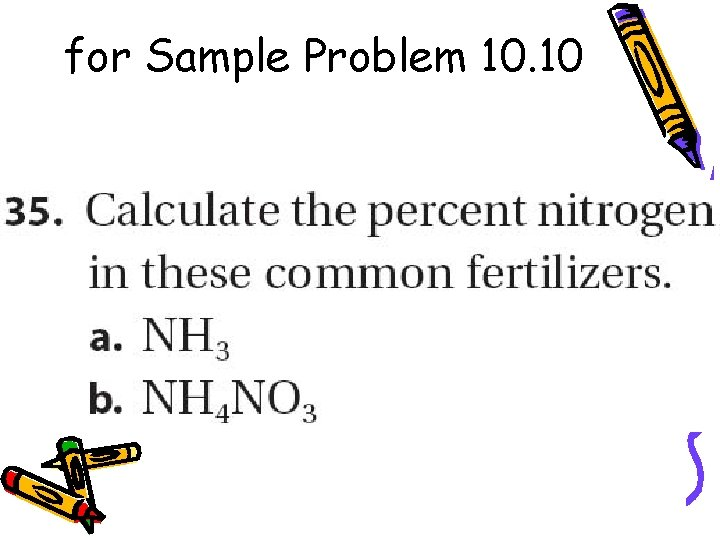for Sample Problem 10. 10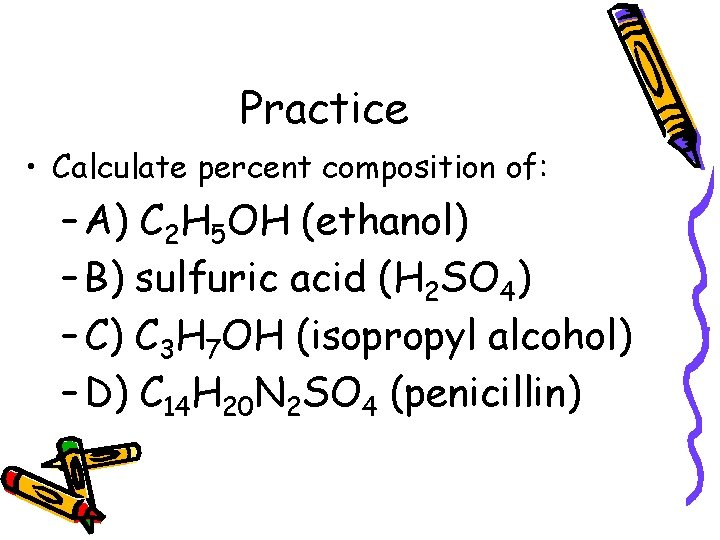Practice • Calculate percent composition of: – A) C 2 H 5 OH (ethanol) – B) sulfuric acid (H 2 SO 4) – C) C 3 H 7 OH (isopropyl alcohol) – D) C 14 H 20 N 2 SO 4 (penicillin)10. 3 Empirical Formulas – The empirical formula gives the lowest whole-number ratio of the atoms of the elements in a compound.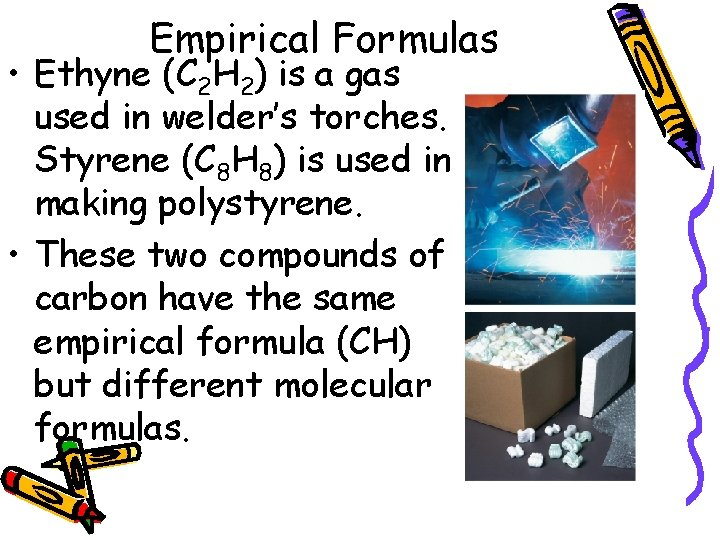10. 3 Empirical Formulas • Ethyne (C 2 H 2) is a gas used in welder’s torches. Styrene (C 8 H 8) is used in making polystyrene. • These two compounds of carbon have the same empirical formula (CH) but different molecular formulas.Write empirical formulas for: • 1) C 5 H 10 O 5 • 2) C 6 H 12 O 2 • 3) C 12 H 17 ON • 4) C 6 H 6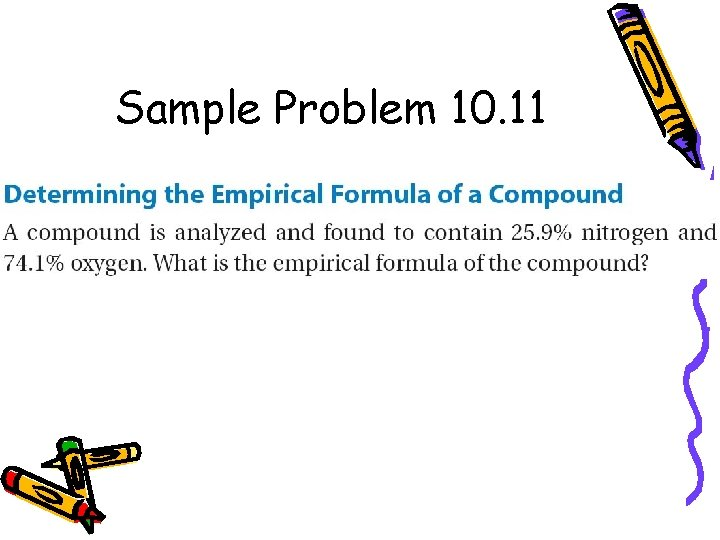Sample Problem 10. 11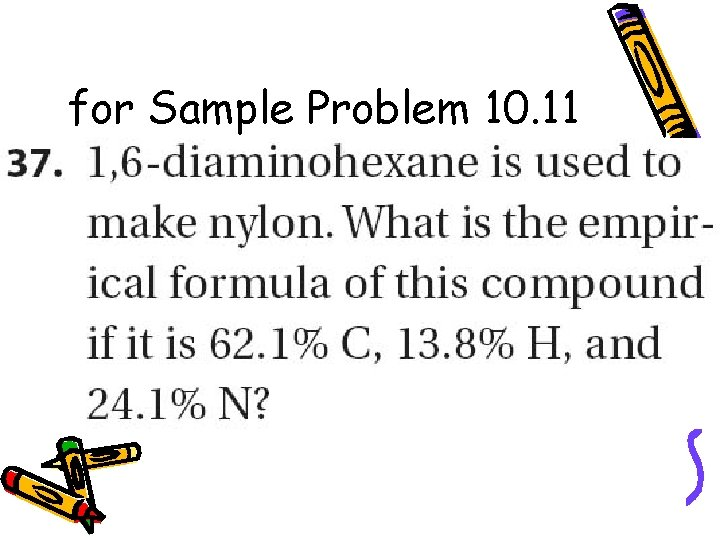for Sample Problem 10. 11Practice – A sample contains 3. 161 g of phosphorus, 0. 3086 g of hydrogen and 6. 531 g of oxygen. Determine the empirical formula.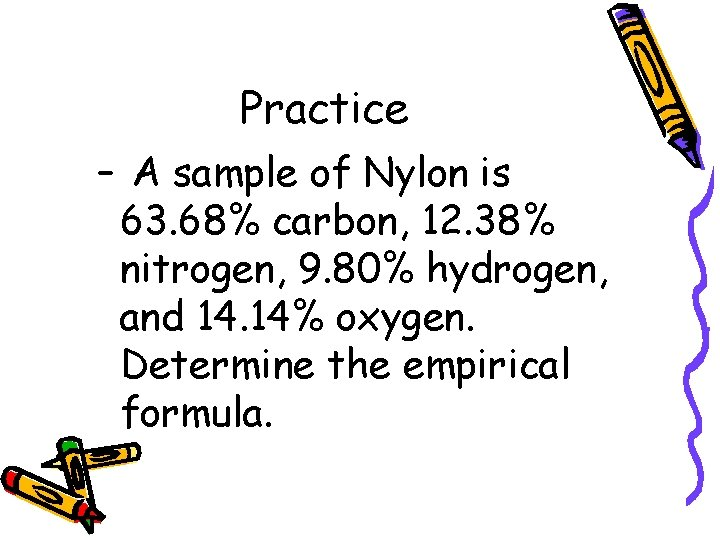Practice – A sample of Nylon is 63. 68% carbon, 12. 38% nitrogen, 9. 80% hydrogen, and 14. 14% oxygen. Determine the empirical formula.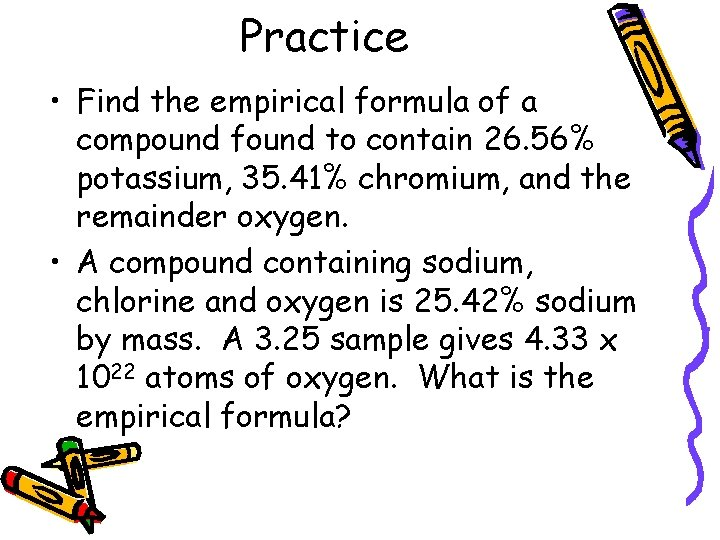Practice • Find the empirical formula of a compound found to contain 26. 56% potassium, 35. 41% chromium, and the remainder oxygen. • A compound containing sodium, chlorine and oxygen is 25. 42% sodium by mass. A 3. 25 sample gives 4. 33 x 1022 atoms of oxygen. What is the empirical formula?10. 3 Molecular Formulas – The molecular formula of a compound is either the same as its experimentally determined empirical formula, or it is a simple whole-number multiple of its empirical formula.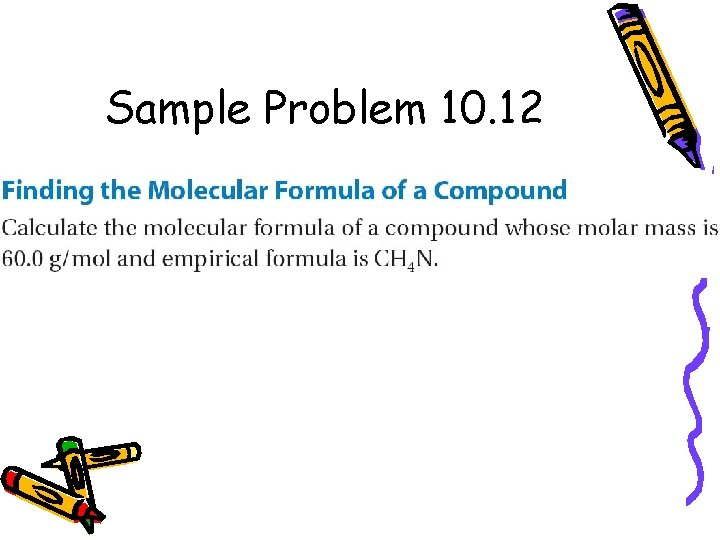Sample Problem 10. 12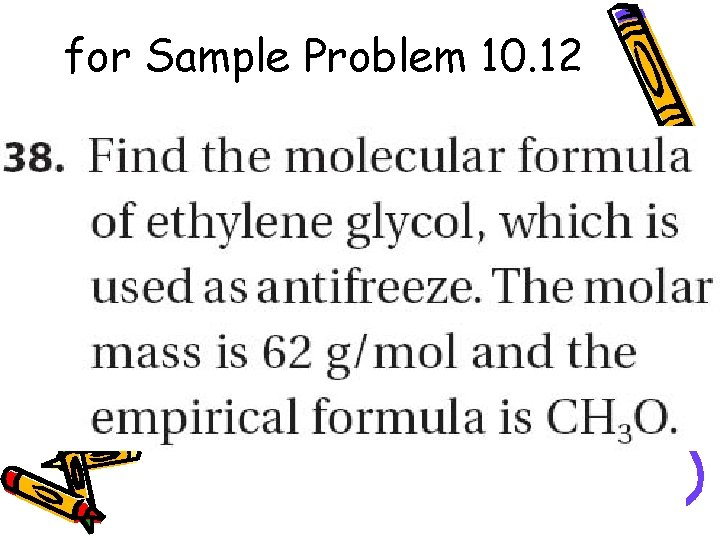for Sample Problem 10. 12Example – A white powder is analyzed and has an empirical formula of P 2 O 5. It has a molar mass of 283. 88 g/mol. What is the molecular formula?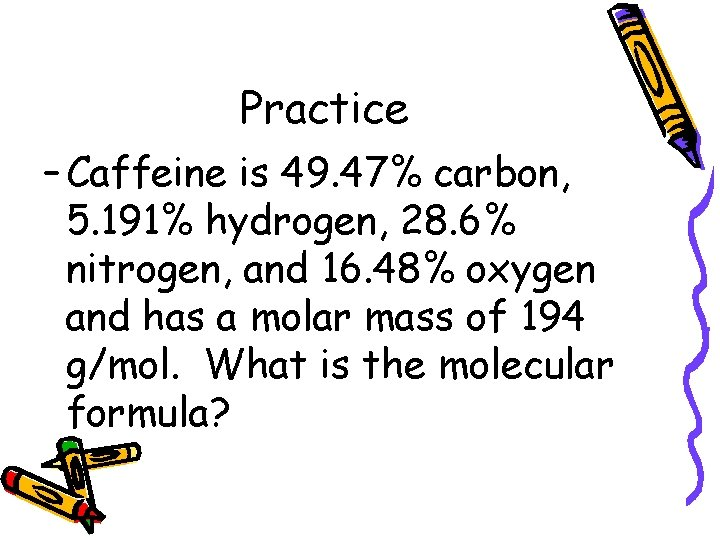Practice – Caffeine is 49. 47% carbon, 5. 191% hydrogen, 28. 6% nitrogen, and 16. 48% oxygen and has a molar mass of 194 g/mol. What is the molecular formula?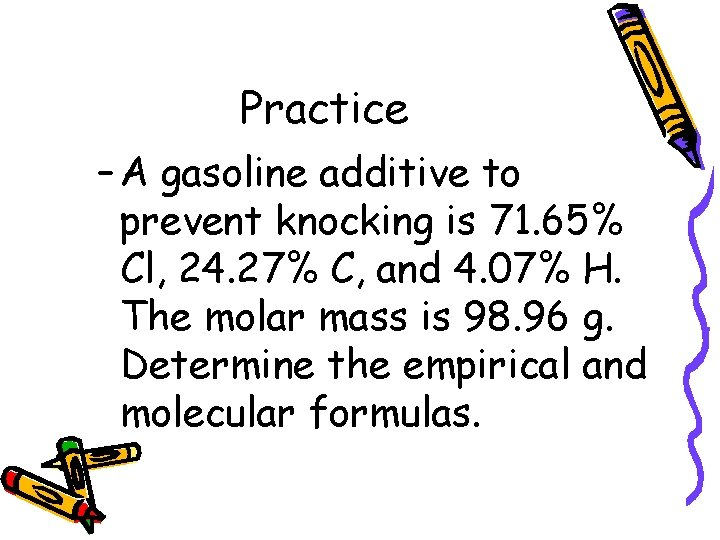Practice – A gasoline additive to prevent knocking is 71. 65% Cl, 24. 27% C, and 4. 07% H. The molar mass is 98. 96 g. Determine the empirical and molecular formulas.10. 3 Section Quiz. – 1. Calculate the percent by mass of carbon in cadaverine, C 5 H 14 N 2, a compound present in rotting meat. a) 67. 4% C b) 58. 8% C c) 51. 7% C d) 68. 2% C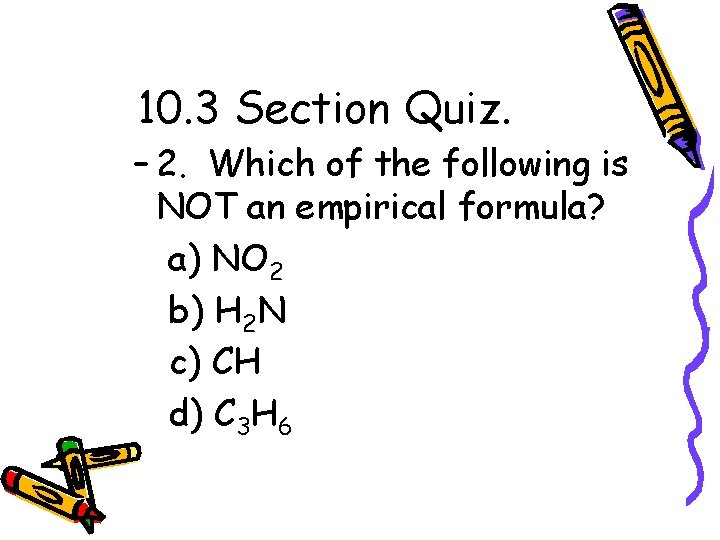10. 3 Section Quiz. – 2. Which of the following is NOT an empirical formula? a) NO 2 b) H 2 N c) CH d) C 3 H 610. 3 Section Quiz. – 3. Determine the molecular formula of a compound that contains 40. 0 percent C, 6. 71 percent H, and 53. 29 percent O and has a molar mass of 60. 05 g. a) C 2 H 4 O 2 b) CH 2 O c) C 2 H 3 O d) C 2 H 4 O